# Formal Verification of ERC20 Contracts

Posted on August 15, 2018 by

Posted in

Runtime Verification Inc provides Formal Smart Contract Verification services.

The previous post explained the overall process of formally verifying a smart contract. It wasn't enough, though, to let you imagine what you'd work with as you did the work. This post expands on the previous one using the recent experience of one of us (Park), who verified several implementations of the ERC-20 standard written to run on the Ethereum Virtual Machine (EVM).

The last post used the following picture to summarize all the pieces of the proving workflow. A contract is formalized in a specification, which is then augmented to describe the behavior of the compiled bytecodes (rather than just the original Solidity source). The bytecodes, bytecode specification, and predefined semantics of the EVM are used by the K framework (together with hints from a human) to determine if the bytecodes match the specification.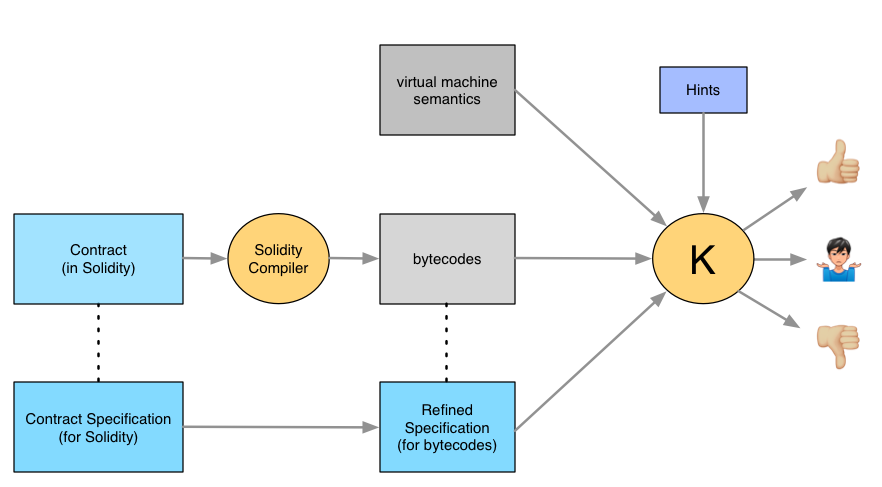To see all the details, including everything you'd need to redo the proofs, see our Github repository.

## The proving process

Because it's hard to read the EVM bytecode assembly language, we'll start by pretending that we have a semantics for the Solidity language and show how proving would then work. That's simpler: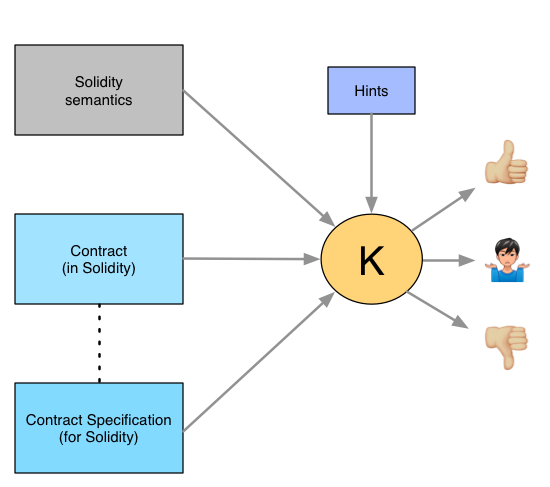Once you understand the simpler case, we'll move on to using bytecodes.

To avoid too much detail, we'll work with the simplest ERC-20 function, `totalSupply`. Here's a correct implementation:

``````uint supply;
function totalSupply() public view returns (uint) {
return supply;
}
``````

That's too simple, so we'll add a bug:

``````function totalSupply() public view returns (uint) {
if (supply == 0x1000000000000000000) {
return 0;
} else {
return supply;
}
}
``````

If you were testing that without knowing the source, you'd be extremely unlikely to find the bug. Let's see how to find it with K. There are four steps:

1. Describe the starting configuration to be verified. In our case it's when `totalSupply()` is called with `supply` having some unspecified value.

2. Ask K to symbolically execute the implementation on that starting configuration, producing some implementation results.

3. Ask K to symbolically execute the specification on the same configuration, producing one or more specification results.

4. Ask K to show that every implementation result is also captured by some specification result. We'll see what that means later. For now, think of it as meaning that the implementation produces all the results required by the specification, but possibly more.

### Step 1: What to check

The actual input to K is a variant of XML:

``````<startWith>
<k>totalSupply()</k>
<supply>SomeSupply</supply>
</startWith>
``````

Each tag (or cell) is defined (elsewhere) to be of some type. The `k` tag is code to be checked. The `supply` tag is an integer.

The content of the `supply` cell is `SomeSupply`. The use of an arbitrary name (rather than an actual integer) means that K isn’t allowed to assume anything about the `supply` other than its type.

(Note: the outer tag isn't actually called `startWith`. The real tag contains information used for other purposes, so we thought it best to simplify here.)

### Step 2: Symbolic execution

Suppose that we, as humans who know Solidity, have to evaluate the situation described with `startWith`. That is, we have to figure out the return value of `totalSupply()` and also any changes it makes to global variables (like `supply`).

The first step is to replace `totalSupply()` with its body:

``````if (supply == 0x1000000000000000000) {
return 0;
} else {
return supply;
}
``````

Now, we hand-execute the body, starting with line 1.

But this is a problem. `startWith` doesn't tell us whether `supply` is `0x1000000000000000000` or not. We have no choice but to compute two results, one for each of two cases:

``````supply == 0x1000000000000000000
supply != 0x1000000000000000000
``````

That's why this technique is called symbolic execution: we can operate on symbols standing in for values, rather than just specific values.

Stepping through the code, we'd quickly produce these two results, written as K would:

``````-- then branch
<symbolicResult>
<k> 0 </k>
<supply> SomeSupply </supply>
</symbolicResult>
requires supply == 0x1000000000000000000

-- else branch
<symbolicResult>
<k> SomeSupply </k>
<supply> SomeSupply </supply>
</symbolicResult>
requires supply != 0x1000000000000000000
``````

The difference between the two cases is whether the result is `0` or the original value of `supply`. The `requires` annotation (lines 6 and 13) describes the circumstances in which the two different results are produced. (Notice that the result is stored in the `k` cell. That will turn out to be important later.)

We've followed the same process as K uses, except that K works from a precise formal semantics rather than our informal understanding of Solidity.

### Step 3: Executing the specification

A K specification contains a number of rules. When given the `startWith` configuration, K's specification executor looks for a rule that matches a `k` cell containing `totalSupply()` and a `supply` cell containing an arbitrary value.

It finds it here:

``````rule <k> totalSupply() => Total ... </k>
<supply> Total </supply>
``````

Notice that the specification uses `Total` instead of `SomeSupply`. The names don't matter to K; each is just a placeholder for some unknown value.

The `k` cell looks different than it did in `startWith`. There's an arrow with `Total` after it. After a rule matches, it fires, meaning that the left-hand side is replaced by the right:

``````<specResult>
<k> Total </k>              -- This changed
<supply> Total </supply>
</specResult>
``````

If there were a rule that matched this new pattern, it would fire, giving us one or more new results. There happens not to be such a rule, so execution stops here.

### Step 4: Comparing the two kinds of results

We now have two symbolic results and one result from the specification. We need to ask if the specification result captures both symbolic results. There are two pairs to check. Here's one:

``````<symbolicResult>                         | <specResult>
<k> SomeSupply </k>                  |   <k> Total </k>
<supply> SomeSupply </supply>        |   <supply> Total </supply>
</symbolicResult>                        | </specResult>
requires supply != 0x1000000000000000000 |
``````

Both sides mean the same thing: the return value is whatever the `supply` is. The fact that one side uses `SomeSupply` to mean "any integer value" and the other uses `Total` doesn't change the fact that they mean the same thing.

So that pair checks out. What about the other one?

``````<symbolicResult>                         | <specResult>
<k> 0 </k>                           |   <k> Total </k>
<supply> SomeSupply </supply>        |   <supply> Total </supply>
</symbolicResult>                        | </specResult>
requires supply == 0x1000000000000000000 |
``````

This is no good. The specification says that the return value is whatever `supply` is, but the symbolic executor has discovered a case where the return value is `0`, no matter what the value of `SomeSupply`.

That's a bug.

## EVM Semantics

We wouldn't find that bug that way, though. In real life, the symbolic executor would work on compiler-generated bytecodes, not Solidity code. And it would know how to execute each bytecode because of rules in the full EVM semantics.

Before looking at the formal semantics, let's take an informal look at how the EVM runs a compiled contract. (You can skip this section if you already know EVM.)

Here's a partial snapshot of the state of the EVM at the moment it executes what was originally `supply == 0x1000000000000000000`: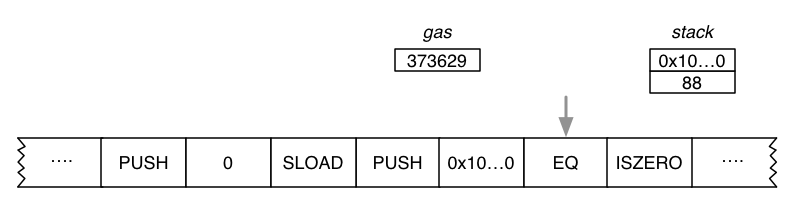The bottom of the picture shows a segment of the EVM bytecodes. The arrow (program counter) points to the `EQ` instruction, which is about to be executed.

The top two elements of the stack (upper right) have been set to `0x1000000000000000000` and the retrieved value of `supply`, which we're assuming is 88. (Remember that we're describing the real EVM, not the symbolic executor, so it has to work with actual – not symbolic – values.)

To the left of the stack, you'll see a record of how much gas remains. EVM transactions have to pay for resources they use. Metaphorically, the creator of a transaction fills it with gas, and the EVM runs the transaction until it's finished or it "runs out of gas."

In the picture, 373629 units of gas remain when `EQ` is to execute. `EQ` costs 3 units, so the gas supply will be 373626 for the next instruction, `ISZERO`.

The stack is the cheapest kind of memory. There are two others of interest: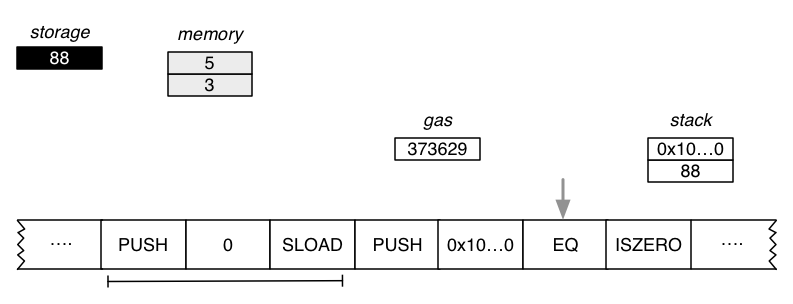The storage is in the upper left. It represents data stored in the blockchain. Such data outlives the transaction that created it, and it can be changed or read by other transactions.

Our original Solidity code used only one storage slot (`supply`), so only one is shown. The value of `supply` was transferred from the storage by the underlined bytecodes in the picture:

1. `PUSH 0` pushes the index of the desired storage slot onto the stack.
2. `SLOAD` retrieves the corresponding storage slot and replaces the top of the stack with it.

Storage is expensive. `SLOAD` costs 200 gas instead of `EQ`'s 3. And changing a storage value costs 20,000 gas.

Stacks are inconvenient for some purposes (like holding values of a higher level language's local variables), so EVM also has memory. Instead of having to get a value to or near the top of a stack before you can use it, all memory can be accessed directly in one step.

`totalSupply` doesn't need memory, but - for illustration - let's pretend it requires two memory slots. (They're shown to the right of storage.)

Instructions that work with memory are approximately ten times as expensive as those that work with the stack, but much cheaper than ones that work with storage. In addition, the transaction is charged gas for the total amount of memory it uses. In this example, the transaction has so far paid for two memory slots.

### EVM semantics in K

K can be used to generate a virtual machine that executes real contracts, or it can symbolically execute a contract. In either case, it must keep track of all the same values that any implementation of EVM must.

Written out in XML-like format, the full EVM state is imposing. Imposing enough, in fact, that we don't want you to read it. But this picture will give you an idea of its size: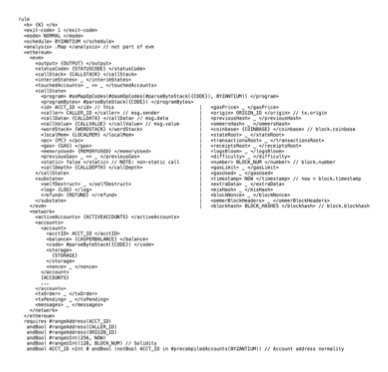Much of that (such as the program being executed) is set once and never changed. For this example, we'll show only what's needed to execute the single bytecode `EQ`. Further, for simplicity, we'll ignore gas calculations, checking for stack overflow or underflow, and similar bookkeeping. We'll also remove some syntax that's not important for your understanding. That leaves us with this starting state: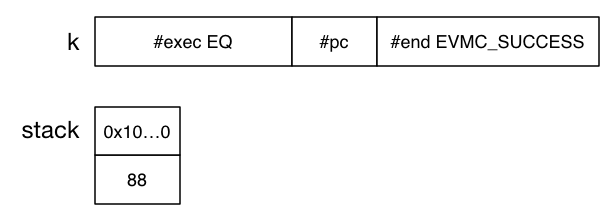The `k` cell is different than we've seen before. In the starting example, it had only one element. Here, it has a list of elements. Rules match the head of the list, and matching continues until the `k` cell is empty.

Here's a rule that matches:

``````rule <k> #exec EQ  => EQ W0 W1  ... </k>
<wordStack> W0 : W1 : WS  => WS </wordStack>

``````

The second line shows the first two words being popped off the stack. Read this as "the stack was originally an element `W0`, followed by an element `W1`, followed by the remainder of the stack `WS` (zero or more elements long). `EQ` starts by transforming the stack to just the remainder."

The first line of the rule shows `#exec EQ` being replaced by `EQ W0 W1`. (The `...` indicates the rest of the `k` cell is left alone.)

After this rule finishes, we have this: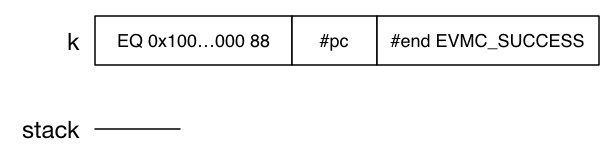(Note: for some reason, the compiler flipped the order of arguments from the original Solidity source. Makes no difference.)

The head of the `k` cell matches this rule:

``````rule <k> EQ W0 W1
=>
W0 ==Word W1 ~> #push
... </k>
``````

That rule replaces the front of the `k` cell with two new elements (often called `terms`):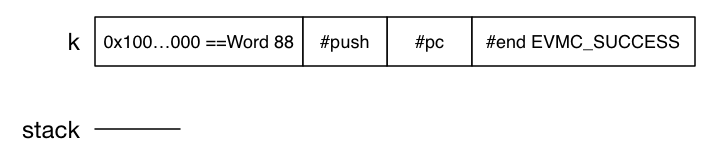(In K code, the `~>` separates the terms.)

The first term (`... ==Word ...`) matches some rules that interpret EVM's 256-bit equality in terms of K's built-in unlimited-precision arithmetic. The difference doesn't matter for equality, but it does matter for operators like `ADD`, where the use of a `+Word` rule causes K to check for 256-bit overflow. (Overflow has been used for exploits like BatchOverflow, which allowed someone to create an absurdly large number of tokens.)

We'll skip those rules and just note that the first term will be replaced by 0 (EVM's representation for `false`):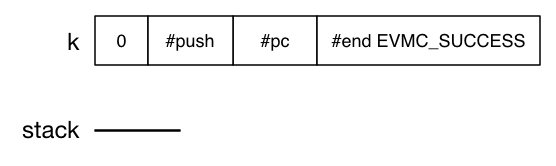Next, a rule that matches two terms fires:

``````    rule <k> W0 ~> #push => . ... </k>
<wordStack> WS => W0 : WS </wordStack>
``````

It puts the result `W0` on the stack and deletes the two terms from `k`, leaving us with this state: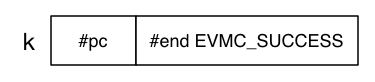Now `#pc` matches a rule (not shown) that initiates the process of advancing the program counter to the next bytecode and instructing K to load it: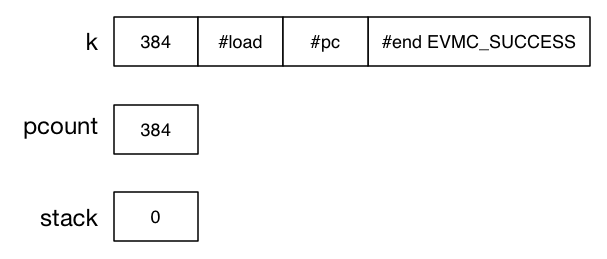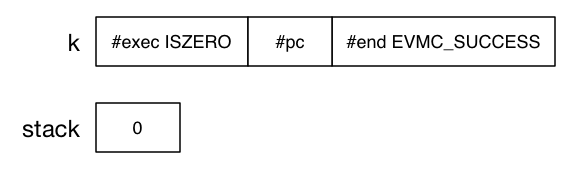... and everything you've just seen starts again.

The process continues until the `#end` term is the only thing left. It matches a rule that records that the execution ended successfully (no stack overflows, arithmetic overflows, gas exceptions, and so on). Note that completing successfully doesn't mean its answer is right. That has to be checked separately.

You can find the EVM specification here. Most of the rules you've seen come from evm.md. data.md shows the nitty-gritty details of, for example, telling K how to convert from the logical notions of `true` and `false` to specific 256-bit integers.

## The proving process, this time for real: EVM

Now let's attempt to prove the compiled version of `totalSupply`, this time from the bytecodes the compiler produces for it.

### Step 1: What to check

The first thing we need to translate to the EVM level is the configuration that both the symbolic executor and the specification will have to work with. In the Solidity version, that was this:

``````<startWith>
<k>totalSupply()</k>
<supply>SomeSupply</supply>
</startWith>
``````

Let's start with `supply`. The following is the way to say "location 0 contains `SomeSupply`":

``````<storage>#hashedLocation(0) |-> SomeSupply</storage>
``````

(As before, some syntax has been removed for simplicity.)

Note that we would have to look at the actual compiler bytecodes (or know its implementation) to know that it puts `supply` at storage location 0.

In the Solidity example, `totalSupply()` was put in `k`. In this example, the compiled version of that call to `totalSupply` will be stored in a cell called `callData`:

``````<callData> #abiCallData("totalSupply", .) </callData>
``````

`#abiCallData` is just a function that translates a function name and, in this case, zero arguments into the format a compiled contract expects to receive.

The `k` cell just holds the term `#execute` to start everything off. The stack and memory are empty.

### Step 2: Symbolic execution

In the explanation of the EVM, we gave a numerical value for `supply`. In this case, `supply` is `SomeSupply`. That means that the `EQ` instruction will result in this comparison: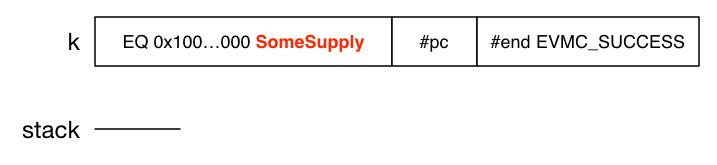As in the Solidity example, symbolic execution must keep track of both possible results for `EQ`. That is, it must maintain two world-states: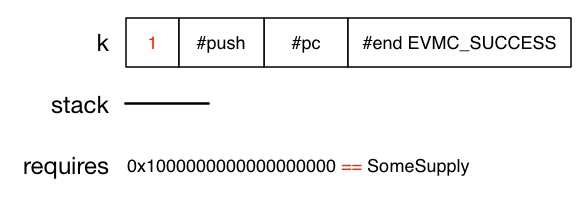and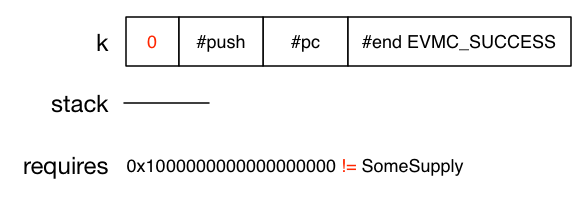Continued execution of the second case will eventually lead to a state we'll draw like this: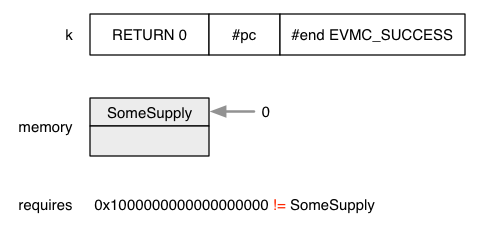The EVM allows multiple return values, all of which are stored in memory. In the case of `totalSupply()`, there's only one return value and no other uses of memory, so the return value is in slot 0. In this result, the slot holds `SomeSupply`.

### Step 3: Executing the specification

Both the symbolic execution engine and the prover work from the same XML-like document, but they treat cells containing `=>` differently. Consider this description of memory:

``````<memory> . => .[ RET_ADDR := SomeSupply ] _ </memory>
``````

The symbolic executor ignores the `=>`. To it, the cell means that `memory` starts empty. Rules matching this and other cells will determine what values it takes on during execution.

To the specification, the cell means "given the starting values the symbolic executor worked with, the end result of `memory` must be described by these facts:

1. There is a memory slot containing `SomeSupply` (which was identified as the only value of `storage` in the initial configuration).

2. The symbolic value `RET_ADDR` labels that memory slot.

3. By the way (says the specification by using `_` at the end of the line), there can be other occupied `memory` slots. Whether there are or not is irrelevant to correctness.

In the `memory` cell, `RET_ADDR` is a free-floating symbol. It's tied down by the neighboring `k` cell:

``````<k>#execute => (RETURN RET_ADDR ~> _)</k>
``````

That says that `k` begins with `RETURN RET_ADDR`. In other words, the argument to `RETURN` identifies a cell that must contain `SomeSupply`.

### Step 4: Comparing the two kinds of results

So the specification requires this:

``````<k>#execute => (RETURN RET_ADDR ~> _)</k>
<memory> . => .[ RET_ADDR := SomeSupply ] _  </memory>
``````

We ended step 2 noting that symbolic execution would create this situation:If you substitute 0 for RET_ADDRESS, you'll see that the symbolic result matches the specification result.

(You may object that, in the Solidity example, we said that the comparison was done after the symbolic execution finished, but here we're comparing it at some seemingly arbitrary step before the end. We, um, lied earlier. The proof is attempted after every step of symbolic execution.)

The symbolic result in the other case will be this: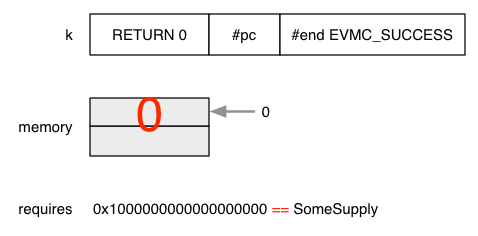Same bug as in the Solidity example.

### Helping the prover

A lot of arithmetic is involved in EVM. Symbolic execution can produce a complicated equation (involving both actual integers and symbolic values) that the prover then has to show is equal to the simpler equation produced from the specification. For some equations, the prover can't do it alone, at least not in anything like a reasonable amount of time.

So a human has to give it rules that help. For example, many humans already know how to find the 50th 8-bit byte in a sequence of 256-byte words. We probably didn't figure it out ourselves – someone told us how. There's no reason to make a prover figure it out, either. We can tell it how, using rules something like this:

``````rule nthbyteof(V, I, N) => nthbyteof(V /Int 256, I, N -Int 1)
requires N  >Int (I +Int 1)

rule nthbyteof(V, I, N) => V %Int 256
requires N ==Int (I +Int 1)
``````

The need for such rules is discovered when K doesn't give an answer for long enough that you decide to find the slowdown and fix it.

For example, reasoning about the MLOAD and MSTORE instructions involves splitting words into bytes and merging bytes into words. That's slow without helper rules like this one:

``````rule #asWord( nthbyteof(V,  0, N) : ... : nthbyteof(V, N -Int 1, N) : .) => V
requires 0 <=Int V
andBool V <Int (2 ^Int (N *Int 8))
andBool 1 <=Int N <=Int 32
``````

## The results

The most exciting ending for this blog post would be us revealing a critical bug in a widely-deployed ERC-20 contract. Sadly for us (but happily for contract users) we didn't.

However, note that one of the contracts we worked with was the HackerGold contract. An earlier version had a spectacular bug that manual audit and testing missed. A simple typo in `transferFrom` meant `=+` was used instead of `+=`. The result was the need to rewrite the contract and scramble to reissue new tokens to everyone who had old ones.

K would have found that, but we thought it unfair to use the version with the known bug. Fortunately for us, it turns out there's still a minor bug in the fixed version of `transferFrom`. Can you find it?

``````function transferFrom(address from, address to, uint256 value) returns (bool success) {
if ( balances[from] >= value &&
allowed[from][msg.sender] >= value &&
value > 0) {

// do the actual transfer
balances[from] -= value;
balances[to] += value;

// addjust the permision, after part of
// permited to spend value was used
allowed[from][msg.sender] -= value;

// rise the Transfer event
Transfer(from, to, value);
return true;
} else {
return false;
}
}
``````

The bug is easier to find if you compare the function to the ERC-20 standard:

transferFrom

Transfers `value` amount of tokens from address `from_`to address `to`, and MUST fire the Transfer event.

The `transferFrom` method is used for a withdraw workflow, allowing contracts to transfer tokens on your behalf. This can be used for example to allow a contract to transfer tokens on your behalf and/or to charge fees in sub-currencies. The function SHOULD throw unless the `from` account has deliberately authorized the sender of the message via some mechanism.

Note Transfers of 0 values MUST be treated as normal transfers and fire the Transfer event.

``````function transferFrom(address from, address to, uint256 value) returns (bool success)
``````

The bug is that the code rejects a transfer of value `0`. If you try it, you get a `false` and no log event. Is that another typo? `>` instead of `>=`? Or is it the unfortunately common event where a programmer assumes some part of the specification is "obvious," doesn't check, so implements the wrong thing?

We don't know. But either case motivates Runtime Verification's goal: make it cost-effective to create a program (like a contract, or a virtual machine) by writing a formal specification and then generating the program with no or little human intervention. As we did with IELE, a different virtual machine for the blockchain.